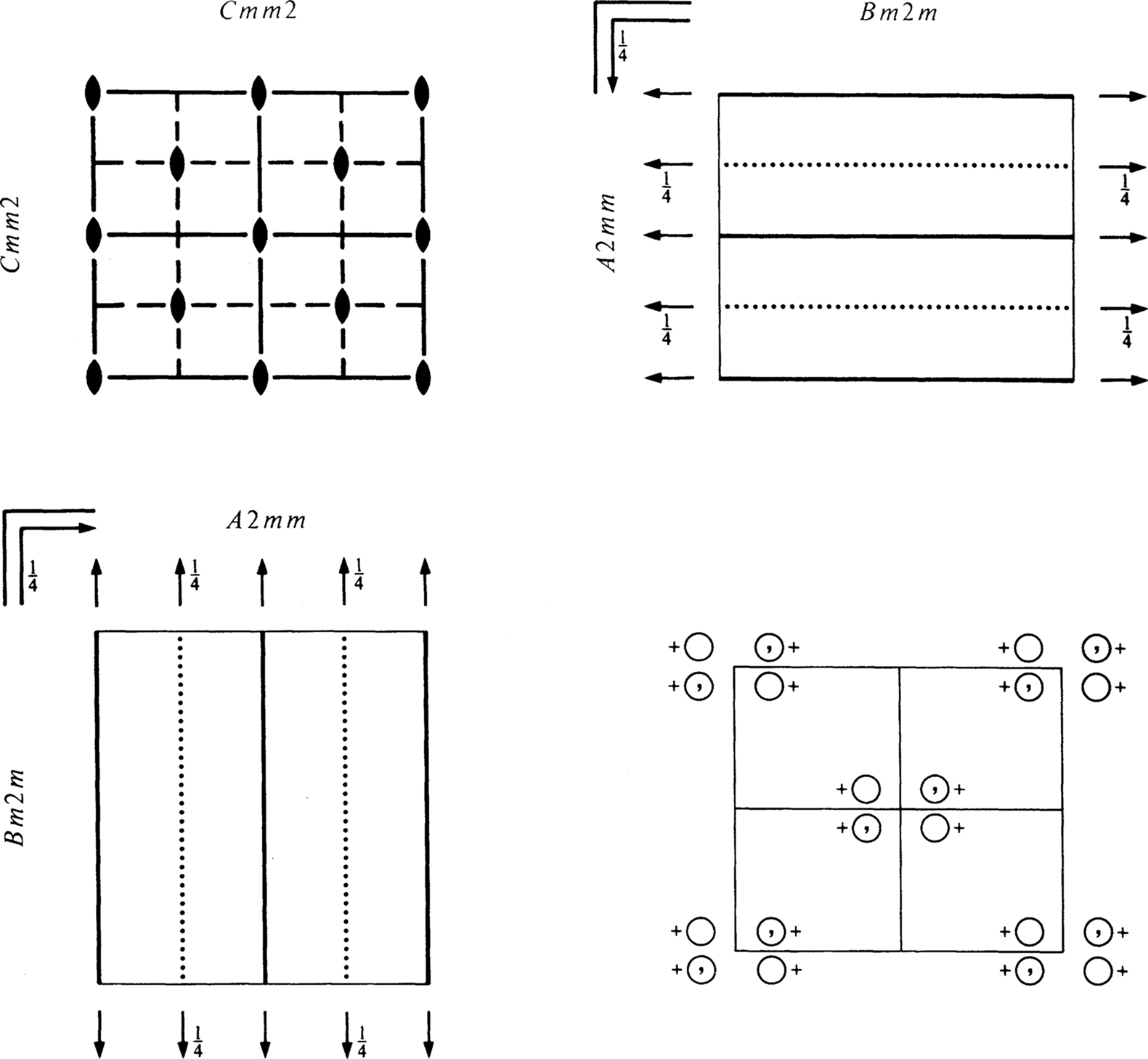Move your mouse over or click on the links to get information about each item. Detailed information on the space-group tables is given in Chapter 2.2.

 Cmm2 C2v11 mm2 Orthorhombic No. 35 Cmm2 Patterson symmetry CmmmOrigin on m m 2

 Asymmetric unit 0 ≤ x ≤ 1/4; 0 ≤ y ≤ 1/2; 0 ≤ z ≤ 1

For (0, 0, 0)+ set

 (1)  1 (2)  2   0, 0, z (3)  m   x, 0, z (4)  m   0, y, z

For (1/21/2, 0)+ set

 (1)  t(1/2, 1/2, 0) (2)  2   1/4, 1/4, z (3)  a   x, 1/4, z (4)  b   1/4, y, z

Generators selected (1); t(1, 0, 0); t(0, 1, 0); t(0, 0, 1); t(1/21/2, 0); (2); (3)

Positions

Multiplicity, Wyckoff letter,
Site symmetry
Coordinates Reflection conditions
(0, 0, 0)+  (1/21/2, 0)+  General:
 8 f 1
 (1) x, y, z (2) -x, -y, z (3) x, -y, z (4) -x, y, z
hkl : h + k = 2n
0kl : k = 2n
h0l : h = 2n
hk0 : h + k = 2n
h00 : h = 2n
0k0 : k = 2n
Special: as above, plus
 4 e m . .
 0, y, z 0, -y, z
no extra conditions
 4 d . m .
 x, 0, z -x, 0, z
no extra conditions
 4 c . . 2
 1/4, 1/4, z 1/4, 3/4, z
hkl : h = 2n
 2 b m m 2
 0, 1/2, z
no extra conditions
 2 a m m 2
 0, 0, z
no extra conditions

Symmetry of special projections

 Along    c2mma' = a   b' = b   Origin at 0, 0, z Along    p1m1a' = 1/2b   b' = c   Origin at x, 0, 0 Along    p11ma' = c   b' = 1/2a   Origin at 0, y, 0

Maximal non-isomorphic subgroups

 I  C1m1 (Cm, 8) (1; 3)+  Cm11 (Cm, 8) (1; 4)+  C112 (P2, 3) (1; 2)+
 IIa  Pba2 (32) 1; 2; (3; 4) + (1/2, 1/2, 0)  Pbm2 (Pma2, 28) 1; 3; (2; 4) + (1/2, 1/2, 0)  Pma2 (28) 1; 4; (2; 3) + (1/2, 1/2, 0)  Pmm2 (25) 1; 2; 3; 4
 IIb  Ima2 (c' = 2c) (46);  Ibm2 (c' = 2c) (Ima2, 46);  Iba2 (c' = 2c) (45);  Imm2 (c' = 2c) (44);  Ccc2 (c' = 2c) (37);  Cmc21 (c' = 2c) (36);  Ccm21 (c' = 2c) (Cmc21, 36)

Maximal isomorphic subgroups of lowest index

 IIc  Cmm2 (c' = 2c) (35);  Cmm2 (a' = 3a or b' = 3b) (35)

Minimal non-isomorphic supergroups

 I  Cmmm (65);  Cmme (67);  P4mm (99);  P4bm (100);  P42cm (101);  P42nm (102);  P-42m (111);  P-421m (113);  P6mm (183)
 II  Fmm2 (42);  Pmm2 (a' = 1/2a, b' = 1/2b) (25)